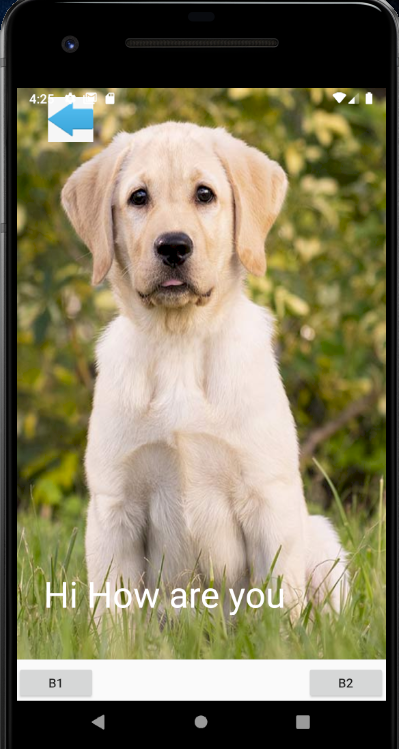## Announcement:

The Xamarin Forums have officially moved to the new Microsoft Q&A experience. Microsoft Q&A is the home for technical questions and answers at across all products at Microsoft now including Xamarin!

To create new threads and ask questions head over to Microsoft Q&A for .NET and get involved today.

# Home Page Design

Hi Guys,
I'm looking same design for Xamarin forms which i attached in the question.
Did any one have idea on this design . Kindly recommended me.

Thanks,
Vasanth

Tagged:

## Answers

• Create a Grid place the image and buttons in the grid Columns. To have the overlapping image or text, Try to use relative layout that could help

• Member, Xamarin Team Xamurai
edited October 2020

As FXamarin suggested , check the implementation

```  <Grid>
<Grid.RowDefinitions>
<RowDefinition Height="*"/>
<RowDefinition Height="40"/>
</Grid.RowDefinitions>

<RelativeLayout BackgroundColor="Red">
<Image Source="a.jpg"
Aspect="Fill"
RelativeLayout.XConstraint="{ConstraintExpression Type=RelativeToParent, Property=X, Constant=0}"
RelativeLayout.YConstraint="{ConstraintExpression Type=RelativeToParent, Property=Y, Constant=0}"
RelativeLayout.WidthConstraint="{ConstraintExpression Type=RelativeToParent, Property=Width, Constant=0}"
RelativeLayout.HeightConstraint="{ConstraintExpression Type=RelativeToParent, Property=Height, Constant=0}"/>
<Image Source="back.png"
RelativeLayout.XConstraint="10"
RelativeLayout.YConstraint="10"
RelativeLayout.WidthConstraint="100"
RelativeLayout.HeightConstraint="50" />
<Label Text="Hi How are you"
FontSize="40"
TextColor="White"
RelativeLayout.XConstraint="30"
RelativeLayout.YConstraint="{ConstraintExpression Type=RelativeToParent, Property=Height, Constant=-100}"
RelativeLayout.WidthConstraint="{ConstraintExpression Type=RelativeToParent, Property=Width, Constant=0}"
RelativeLayout.HeightConstraint="50"/>
</RelativeLayout>
<StackLayout Orientation="Horizontal" Grid.Row="1">
<Button HorizontalOptions="StartAndExpand" Text="B1"/>
<Button HorizontalOptions="EndAndExpand" Text="B2"/>
</StackLayout>
</Grid>
```• Thanks for your valuable result . I'm looking for the page must scroll and the post will be different images . Is that possible ?

• Member, Xamarin Team Xamurai
edited October 2020

Wrap the RelativeLayout into ScrollView .

Or Use CarouselView instead .

• <Grid.RowDefinitions>

</Grid.RowDefinitions>

```<ScrollView BackgroundColor="Red">
<Image Source="a.jpg"
Aspect="Fill"
RelativeLayout.XConstraint="{ConstraintExpression Type=RelativeToParent, Property=X, Constant=0}"
RelativeLayout.YConstraint="{ConstraintExpression Type=RelativeToParent, Property=Y, Constant=0}"
RelativeLayout.WidthConstraint="{ConstraintExpression Type=RelativeToParent, Property=Width, Constant=0}"
RelativeLayout.HeightConstraint="{ConstraintExpression Type=RelativeToParent, Property=Height, Constant=0}"/>
<Image Source="back.png"
RelativeLayout.XConstraint="10"
RelativeLayout.YConstraint="10"
RelativeLayout.WidthConstraint="100"
RelativeLayout.HeightConstraint="50" />
<Label Text="Hi How are you"
FontSize="40"
TextColor="White"
RelativeLayout.XConstraint="30"
RelativeLayout.YConstraint="{ConstraintExpression Type=RelativeToParent, Property=Height, Constant=-100}"
RelativeLayout.WidthConstraint="{ConstraintExpression Type=RelativeToParent, Property=Width, Constant=0}"
RelativeLayout.HeightConstraint="50"/>
</ScrollView >
<StackLayout Orientation="Horizontal" Grid.Row="1">
<Button HorizontalOptions="StartAndExpand" Text="B1"/>
<Button HorizontalOptions="EndAndExpand" Text="B2"/>
</StackLayout>
```

Is this correct ?

• Member, Xamarin Team Xamurai

Something like this

``` <ScrollView>
<RelativeLayout BackgroundColor="Red">
<Image Source="a.jpg"
Aspect="Fill"
RelativeLayout.XConstraint="{ConstraintExpression Type=RelativeToParent, Property=X, Constant=0}"
RelativeLayout.YConstraint="{ConstraintExpression Type=RelativeToParent, Property=Y, Constant=0}"
RelativeLayout.WidthConstraint="{ConstraintExpression Type=RelativeToParent, Property=Width, Constant=0}"
RelativeLayout.HeightConstraint="{ConstraintExpression Type=RelativeToParent, Property=Height, Constant=0}"/>
<Image Source="back.png"
RelativeLayout.XConstraint="10"
RelativeLayout.YConstraint="10"
RelativeLayout.WidthConstraint="100"
RelativeLayout.HeightConstraint="50" />
<Label Text="Hi How are you"
FontSize="40"
TextColor="White"
RelativeLayout.XConstraint="30"
RelativeLayout.YConstraint="{ConstraintExpression Type=RelativeToParent, Property=Height, Constant=-100}"
RelativeLayout.WidthConstraint="{ConstraintExpression Type=RelativeToParent, Property=Width, Constant=0}"
RelativeLayout.HeightConstraint="50"/>
</RelativeLayout>
</ScrollView>
```
• actually i added one scroll view inside the two relative layout . Its not working . Now its basically static page . Later i will bind into database. if i bind i have more than 100 post i like . how i will achieve like facebook or instagram post

Sign In or Register to comment.